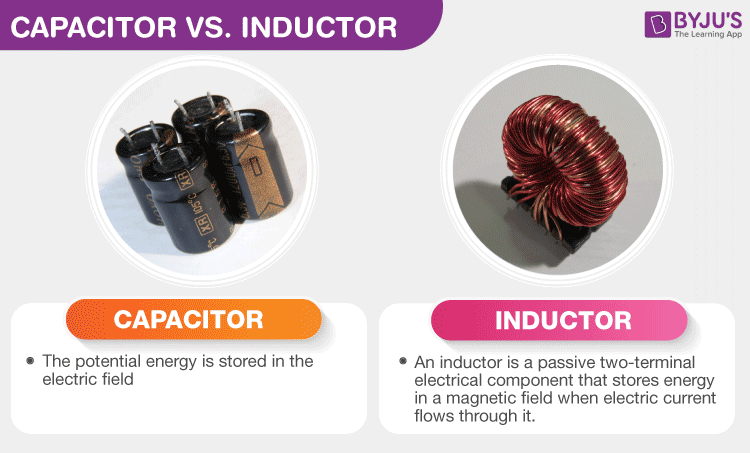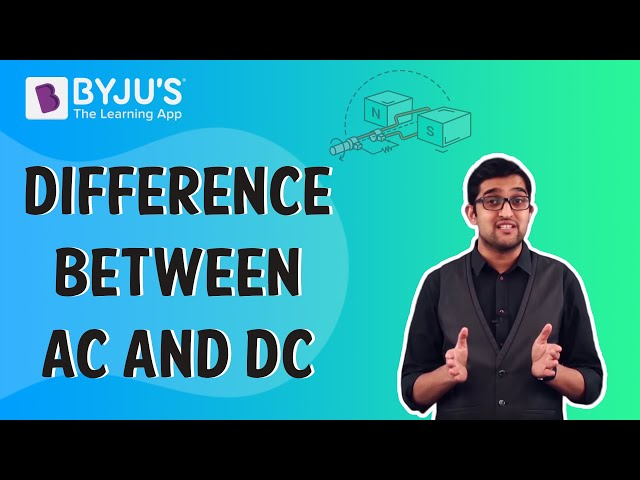Checkout JEE MAINS 2022 Question Paper Analysis : Checkout JEE MAINS 2022 Question Paper Analysis :

# Difference Between Capacitor And Inductor

One of the main differences between a capacitor and an inductor is that a capacitor opposes a change in voltage while an inductor opposes a change in the current. Furthermore, the inductor stores energy in the form of a magnetic field, and the capacitor stores energy in the form of an electric field. In this article, learn more differences between inductors and capacitors.## Capacitor vs Inductor

 Capacitor Inductor It resists changes in voltage It resists changes in current The voltage in a capacitor doesn’t change instantaneously The current in an inductor doesn’t change instantaneously The unit of capacitance is Farad The unit of inductance is Henry Voltage lags current by π/2 Current lags voltage by π/2 The capacitor functions as a short circuit for alternating current Inductor functions as a short circuit for direct current Energy is stored in the electric field Energy is stored in the magnetic field

The listed were a few differences between inductors and capacitors. Both these electrical components impede the flow of electrons in a circuit. Unlike resistors that dissipate energy, capacitors and inductors store energy in an electric field and magnetic field respectively.

## Watch the video and explore the difference between AC and DC.At BYJU’S learn more differences like the difference between diode and rectifier.

Test your knowledge on Capacitor and inductor difference